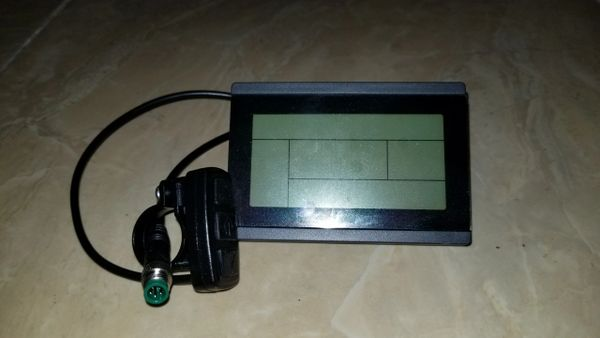\$75.99

This item is out of stock

KT-LCD3 with USB for the Rad bikes, now available ready to ship. It's plug and play to our 25a & 35a controller (Rad) which is required to use with this LCD. Please see link below for the 35a controller (Rad).

Use these LCD settings for the Rad bike and watch the video link below on how to get into the settings.

P1 = 100

P2 = 5

P3 = 0

P4 = 0

P5 = 15

C1 = 3

C2 = 0

C3 = 8

C4 = 3

C5 = 10

C6 = 3

C7 = 1

C8 = 0

C9 = 0

C10 = n

C11 = 0

C12 = 4

C13 = 0

C14 = 2